# Average Ignore 0 – Excel & Google Sheets

This tutorial will demonstrate how to calculate the average of a set of numbers while ignoring zero values in Excel and Google Sheets.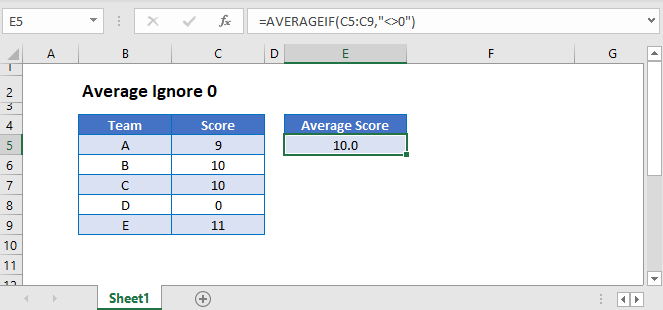## The AVERAGE Functions

The AVERAGE Function calculates the average of a set of numbers, including zeros. To calculate the average, ignoring zeros, you can use the AVERAGEIF Function.

## Ignore Zero Values With AVERAGEIF Function

This example calculates the Average Score of 5 teams while ignoring Scores with a value of 0:

``=AVERAGEIF(C3:C7,"<>0")``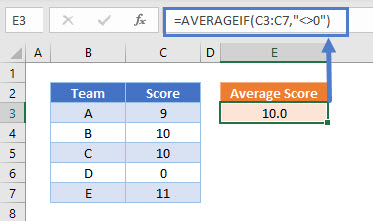## Ignore Text and Blank Values with AVERAGE Function

The AVERAGE and AVERAGEIF Functions ignore text and blank values.

This example demonstrates this::

``=AVERAGE(C3:C7)``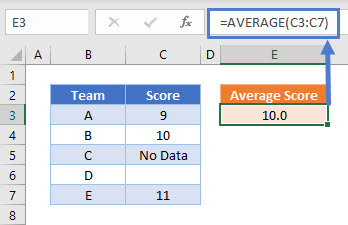## Treat Text Values as Zero With AVERAGEA Function

In order to treat text values as zero in the calculation of the average of a list of values, the AVERAGEA Function can be used:

``=AVERAGEA(C3:C7)``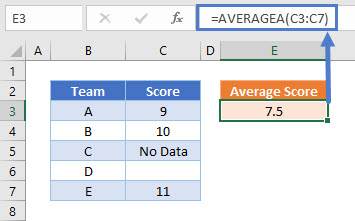As with the AVERAGE and AVERAGEIF Functions, blank cells are ignored by the AVERAGEA Function

## Ignore Zero Values in Google Sheets

These formulas work in exactly the same way in Google Sheets as in Excel.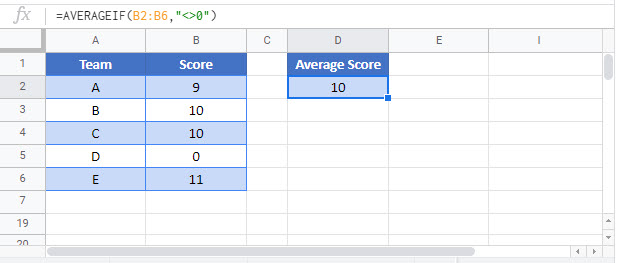### Excel Practice Worksheet

Practice Excel functions and formulas with our 100% free practice worksheets!